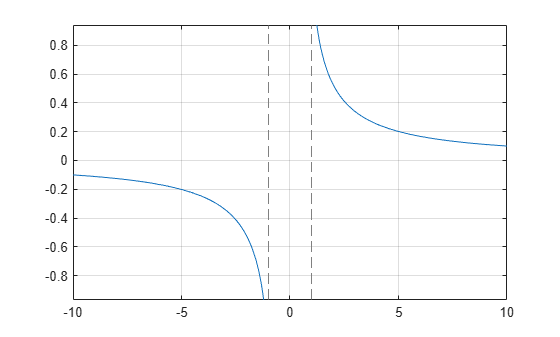# acsc

Symbolic inverse cosecant function

## Syntax

``acsc(X)``

## Description

example

````acsc(X)` returns the inverse cosecant function (arccosecant function) of `X`. All angles are in radians. For real values of `X` in intervals `[-Inf,-1]` and `[1,Inf]`, `acsc` returns real values in the interval `[-pi/2,pi/2]`.For real values of `X` in the interval `[-1,1]` and for complex values of `X`, `acsc` returns complex values with the real parts in the interval `[-pi/2,pi/2]`. ```

## Examples

### Inverse Cosecant Function for Numeric and Symbolic Arguments

Depending on its arguments, `acsc` returns floating-point or exact symbolic results.

Compute the inverse cosecant function for these numbers. Because these numbers are not symbolic objects, `acsc` returns floating-point results.

`A = acsc([-2, 0, 2/sqrt(3), 1/2, 1, 5])`
```A = -0.5236 + 0.0000i 1.5708 - Infi 1.0472 + 0.0000i 1.5708... - 1.3170i 1.5708 + 0.0000i 0.2014 + 0.0000i```

Compute the inverse cosecant function for the numbers converted to symbolic objects. For many symbolic (exact) numbers, `acsc` returns unresolved symbolic calls.

`symA = acsc(sym([-2, 0, 2/sqrt(3), 1/2, 1, 5]))`
```symA = [ -pi/6, Inf, pi/3, asin(2), pi/2, asin(1/5)]```

Use `vpa` to approximate symbolic results with floating-point numbers:

`vpa(symA)`
```ans = [ -0.52359877559829887307710723054658,... Inf,... 1.0471975511965977461542144610932,... 1.5707963267948966192313216916398... - 1.3169578969248165734029498707969i,... 1.5707963267948966192313216916398,... 0.20135792079033079660099758712022]```

### Plot Inverse Cosecant Function

Plot the inverse cosecant function on the interval from -10 to 10.

```syms x fplot(acsc(x),[-10 10]) grid on```### Handle Expressions Containing Inverse Cosecant Function

Many functions, such as `diff`, `int`, `taylor`, and `rewrite`, can handle expressions containing `acsc`.

Find the first and second derivatives of the inverse cosecant function:

```syms x diff(acsc(x), x) diff(acsc(x), x, x)```
```ans = -1/(x^2*(1 - 1/x^2)^(1/2)) ans = 2/(x^3*(1 - 1/x^2)^(1/2)) + 1/(x^5*(1 - 1/x^2)^(3/2))```

Find the indefinite integral of the inverse cosecant function:

`int(acsc(x), x)`
```ans = x*asin(1/x) + log(x + (x^2 - 1)^(1/2))*sign(x)```

Find the Taylor series expansion of `acsc(x)` around ```x = Inf```:

`taylor(acsc(x), x, Inf)`
```ans = 1/x + 1/(6*x^3) + 3/(40*x^5)```

Rewrite the inverse cosecant function in terms of the natural logarithm:

`rewrite(acsc(x), 'log')`
```ans = -log(1i/x + (1 - 1/x^2)^(1/2))*1i```

## Input Arguments

collapse all

Input, specified as a symbolic number, variable, expression, or function, or as a vector or matrix of symbolic numbers, variables, expressions, or functions.